Understanding Nodes

XPath models an XML document as a tree of nodes . This way of looking at an XML document is called XPath's data model. Different types of nodes are available in XPath, such as element nodes, attribute nodes, and text nodes, and we're going to take a look at the various possibilities now.

XPath Node Types

There are seven types of nodes in XPath 1.0:

• Root nodes

• Element nodes

• Attribute nodes

• Processing instruction nodes

• Comment nodes

• Text nodes

• Namespace nodes

We'll take a look at each of these node types here, using our XML document that holds planetary data, renumbered ch02_01.xml for this chapter, as you can see in Listing 2.1.

Listing 2.1 Our Sample XML Document ( ch02_01.xml )
<?xml version="1.0"?> <planets>     <planet>         <name>Mercury</name>         <mass units="(Earth = 1)">.0553</mass>         <day units="days">58.65</day>         <radius units="miles">1516</radius>         <density units="(Earth = 1)">.983</density>         <distance units="million miles">43.4</distance>         <!--At perihelion-->     </planet>     <planet>         <name>Venus</name>         <mass units="(Earth = 1)">.815</mass>         <day units="days">116.75</day>         <radius units="miles">3716</radius>         <density units="(Earth = 1)">.943</density>         <distance units="million miles">66.8</distance>         <!--At perihelion-->     </planet>     <planet>         <name>Earth</name>         <mass units="(Earth = 1)">1</mass>         <day units="days">1</day>         <radius units="miles">2107</radius>         <density units="(Earth = 1)">1</density>         <distance units="million miles">128.4</distance>         <!--At perihelion-->     </planet> </planets>

We'll begin with the root node.

The Root Node

The root node is the root of the XPath tree for an XML document. This node is not the same as the <planets> element in ch02_01.xml <planets> is the document element for the XML document, and people often confuse the two.

The root node is really a logical node that serves simply as the root of the whole XPath node tree. The root node gives you access to the whole tree, and in XPath, you use / to stand for the root node. When you use an XPath expression like /planets , you're starting at the root node and searching for <planets> elements that are direct children of the root node. In fact, you can see this XPath expression at work in our XML document in Figure 2.1 in the XPath Visualiser, as we first saw in Chapter 1.

Figure 2.1. The <planets> child of the root node.Because the root node is the root of the XPath tree, the root node is the same as the entire document, as far as many applications go. Note also that the root node includes not only the document element (and therefore all its children as well), but also any processing instructions, namespace declarations, and so on that are at the same level as the document element.

Element Nodes

We're already familiar with element nodes because they correspond to the elements in an XML documentthere is one element node in the XPath node tree for every element in the original XML document. You can see plenty of elements in our sample XML document, ch02_01.xml , such as <planets> , <planet> , and so on:

<?xml version="1.0"?> <planets>     <planet>         <name>Mercury</name>         <mass units="(Earth = 1)">.0553</mass>         <day units="days">58.65</day>         <radius units="miles">1516</radius>         <density units="(Earth = 1)">.983</density>         <distance units="million miles">43.4</distance>         <!--At perihelion-->     </planet>         .         .         .

Element nodes can also have children, of course. The children of each element node can include element nodes, comment nodes, processing instruction nodes, and text nodes.

Element nodes can also have a unique identifier (ID). For example, if the XML document has an attribute declared to be of type ID, that attribute can serve as the element's ID value. On the other hand, if you do not declare any attributes to be of type ID, no elements can have IDs.

In XPath, you can use an element's name (such as planet for the <planets> element) to match an element, or * to match any element. For example, you can see the XPath expression //* at work in Figure 2.2, matching all element nodes in ch02_01.xml .

Figure 2.2. Matching element nodes.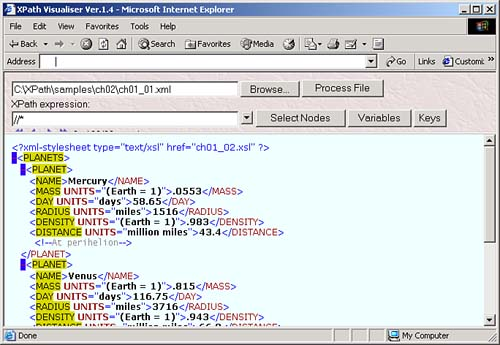Note that if you use an expression such as /planet , you'll get not only a <planet> element (if there is one), but also all its contents. Take a look at this example:

<planet>     <name>Mercury</name>     <mass units="(Earth = 1)">.0553</mass> </planet>

In this case, /planet will return the <planet> element, which includes all that element's contents. In other words, what you get includes a newline character, some whitespace, the <name> element, another newline character, and some additional whitespace, the <mass> element, and a newline character. So the entire element and all its contents are returned. (As we'll see in Chapter 4, you can suppress leading and trailing whitespace with the normalize-space function.)

Attribute Nodes

We're already familiar with attribute nodes because they correspond to element attributes in XML. For example, this element in ch02_01.xml has an attribute named units with the value "days":

<?xml version="1.0"?> <planets>     <planet>         <name>Mercury</name>         <mass units="(Earth = 1)">.0553</mass>  <day units="days">58.65</day>  <radius units="miles">1516</radius>         <density units="(Earth = 1)">.983</density>         <distance units="million miles">43.4</distance>         <!--At perihelion-->     </planet>         .         .         .

Elements can have more than one attribute, of course, and therefore more than one attribute node:

In XPath terms, the element is the parent of each of its attribute nodeshowever, an attribute node is not considered a child of its parent element. Note that this is different from the W3C XML Document Object Model (DOM), which does not treat the element with an attribute as the parent of the attribute.

In XML, you can also have default attributes , where attributes are given default values. For example, some attributes, like xml:lang and xml:space , affect all elements that are descendants of the element with the attributebut that does not affect where attribute nodes appear in the tree. These attributes, like any other, are only considered attributes of their parent elements in XPath.

In XPath, you can refer to attributes using the attribute axis or its shorthand version, @ . For example, to recover the value of the units attribute for an element, you can use the term @units , as we've seen in Chapter 1. To match all attributes in a document, you can use the XPath expression //@* , and you can see that expression at work on ch02_01.xml in the XPath Visualiser in Figure 2.3.

Figure 2.3. Matching attribute nodes.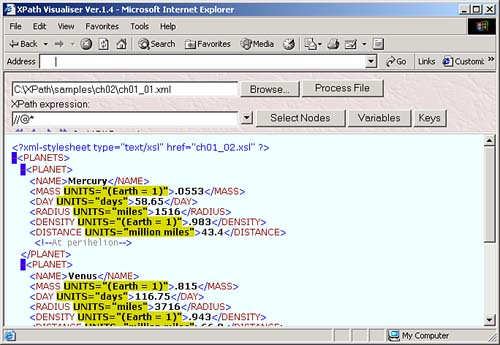NO ATTRIBUTE NODES FOR NAMESPACE ATTRIBUTES

Bear in mind, however, that there are no attribute nodes in XPath corresponding to attributes that declare namespaces.

Processing Instruction Nodes

There is a processing instruction node for every XML processing instruction. For example, there's a processing instruction in ch02_01.xml , <?xml-stylesheet?> , which looks like this:

<?xml version="1.0"?>  <?xml-stylesheet type="text/xsl" href="ch01_02.xsl"?>  <planets>     <planet>         <name>Mercury</name>         .         .         .

Processing instructions are not under the control of any namespace, so they do not have namespace nodes. Also, in XML, their attributes are really pseudo-attributes , which means that XPath will not recognize them as attributes. From an XPath 1.0 point of view, the value of a processing instruction is everything following the processing instruction's target ( xml-stylesheet here) up to the final ? . For example, the value of <?xml-stylesheet type="text/xsl" href="ch01_02.xsl"?> is type="text/xsl" href="ch01_02.xsl" .

THE XML DECLARATION IS NOT A PROCESSING INSTRUCTION

It's important to realize that the XML declaration is not a processing instruction. That means that there is no processing instruction node corresponding to the XML declaration.

You can use the processing-instruction node test to match processing instructions in XPath, which means that you can match all processing instructions in a document with the expression //processing-instruction() as you can see in Figure 2.4.

Figure 2.4. Matching processing instruction nodes.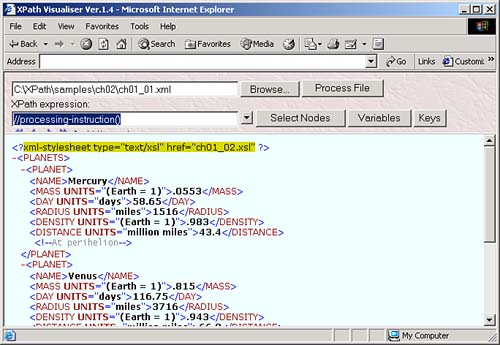ACCESSING A PROCESSING INSTRUCTION'S PSEUDO-ATTRIBUTES

Although you can't directly address the value of a processing instruction's pseudo-attributes using XPath, you can use the string-handling functions we'll see in Chapter 4 to get their values.

Comment Nodes

As you'd expect, comment nodes in XPath correspond to comments in XML documents, which are delimited with <!-- and --> . As far as XPath is concerned , the value of a comment node is the text between <!-- and --> . In an XPath document tree, there is a comment node for every comment (except for any comment that occurs in a DTD or schema).

Our XML document contains a few comments, and you can see one of them here:

<?xml version="1.0"?> <planets>     <planet>         <name>Mercury</name>         <mass units="(Earth = 1)">.0553</mass>         <day units="days">58.65</day>         <radius units="miles">1516</radius>         <density units="(Earth = 1)">.983</density>         <distance units="million miles">43.4</distance>  <!--At perihelion-->  </planet>         .         .         .

In XPath, you can match comments with the comment node test, which means that the expression //comment() matches all comment nodes in a document. You can see this expression at work in the XPath Visualiser in Figure 2.5, where it is matching comment nodes.

Figure 2.5. Matching comment nodes.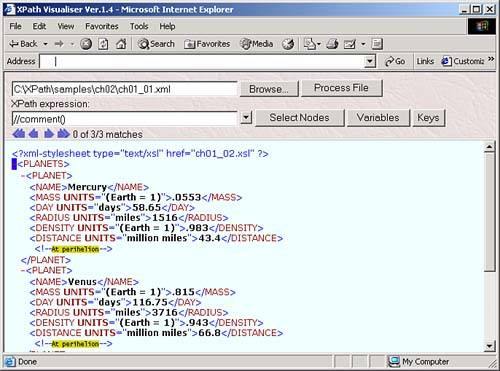Text Nodes

XPath also gives you the means of handling text data in elements as text nodes . For example, the value of the text node in the <name> element here is "Mercury":

<?xml version="1.0"?> <planets>     <planet>  <name>Mercury</name>  <mass units="(Earth = 1)">.0553</mass>         <day units="days">58.65</day>         <radius units="miles">1516</radius>         <density units="(Earth = 1)">.983</density>         <distance units="million miles">43.4</distance>         <!--At perihelion-->     </planet>         .         .         .

A text node of an element is just the PCDATA data of that element. Note that if an element contains other elements, processing instructions, or comments, that can break up text into multiple text nodes. For example, the element <planet>Mars<HR/>The Red Planet</planet> contains two text nodes, "Mars" and "The Red Planet".

HANDLING TEXT IN XML CDATA SECTIONS

How does XPath handle text in XML CDATA sections? Each character within a CDATA section is treated as character data. In other words, a CDATA section is treated as if the <![CDATA[ and ]]> were removed and every occurrence of markup like < and & was replaced by the corresponding character entities like &lt; and &amp; .

Also, characters inside comments, processing instructions, and attribute values do not produce text nodes.

In XPath, you can match text nodes with the text node function, which means that you can match all text nodes throughout a document with the expression //text() , as you see in the XPath Visualiser in Figure 2.6.

Figure 2.6. Matching text nodes.Namespace Nodes

Namespace nodes are a little different from other nodesthey're not visible in the same way in a document. Each element has a set of namespace nodes, one for each distinct namespace prefix that is in scope for the element (including the standard XML prefix, which is implicitly declared by the XML Namespaces Recommendation) and one for the default namespace if one is in scope for the element. The element itself is the parent of each of these namespace nodes; however, a namespace node is not considered a child of its parent element. An element will have a namespace node

• For every attribute in the element that declares a namespace (that is, whose name starts with xmlns: ).

• For every attribute in a containing element whose name starts xmlns: (unless the element itself or a nearer ancestor redeclares the prefix).

• For an xmlns attribute, if the element or some containing element has an xmlns attribute, and the value of the xmlns attribute for the nearest such element is not empty.

Namespace nodes are not directly visible in an XML document, so there's no XPath Visualiser example here. But take a look at this XSLT stylesheet, which includes two explicit namespace declarations:

<?xml version="1.0"?>  <xsl:stylesheet version="1.0"   xmlns:xsl="http://www.w3.org/1999/XSL/Transform"   xmlns="http://www.w3.org/1999/xhtml">  <xsl:template match="//planets">         <html>             <xsl:apply-templates/>         </html>     </xsl:template>     <xsl:variable name="myPosition" select="3"/>     <xsl:template match="planet">         <p>             <xsl:value-of select="\$myPosition"/>          </p>     </xsl:template> </xsl:stylesheet>

In this case, the prefix xsl is associated with the URI "http://www.w3.org/1999/XSL/Transform", and any elements whose names are prefixed with xsl will have a namespace node with the value "http://www.w3.org/1999/XSL/Transform". There's also a default namespace here, "http://www.w3.org/1999/xhtml", used for any non-prefixed elements. And there's another default namespace here, the implicit XML namespace, which is in effect for all XML elements. The URI for the implicit XML namespace is "http://www.w3.org/XML/1998/namespace".

That completes our overview of the seven types of nodes in XPath 1.0: root nodes, element nodes, attribute nodes, processing instruction nodes, comment nodes, text nodes, and namespace nodes. However, there's more about nodes to understand from XPath's point of viewnodes can also have various kinds of names, as well as string values, for example.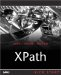XPath Kick Start: Navigating XML with XPath 1.0 and 2.0
ISBN: 0672324113
EAN: 2147483647
Year: 2002
Pages: 131

Similar book on Amazon